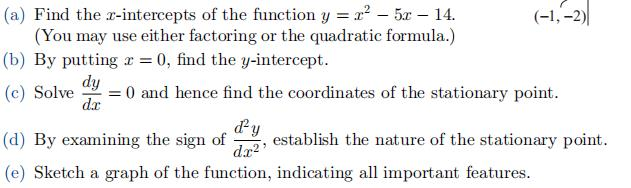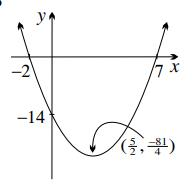### Still have math questions?

Algebra
Question(a) Find the $$x$$ -intercepts of the function $$y = x ^ { 2 } - 5 x - 14 .$$

(You may use either factoring or the quadratic formula.) (b) By putting $$x = 0$$ , find the $$y$$ -intercept. (c) Solve $$\frac { d y } { d x } = 0$$ and hence find the coordinates of the stationary point. (d) By examining the sign of $$\frac { d ^ { 2 } y } { d x ^ { 2 } }$$ , establish the nature of the stationary point. (e) Sketch a graph of the function, indicating all important features.